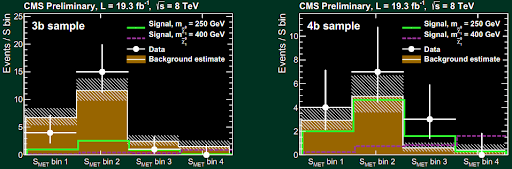## Wednesday, February 19, 2014

### CMS reports a slight higgsino excess: no exclusions possible

The CMS collaboration at the LHC has released two new papers today (so far). One of them reinterprets some results on long-lived charged particles in pMSSM. The other one is arguably more interesting:
Search for electroweak production of higgsinos in channels with two Higgs bosons decaying to $$b$$ quarks in $$pp$$-collisions at $$8\TeV$$
It is based on $$19.3/{\rm fb}$$ of the 2012 data. They wanted to exclude the higgsinos with masses between $$270$$ and $$350\GeV$$. However, the exclusions couldn't be derived due to a "slight excess" of the relevant events. This is a seemingly exciting and surely unusual conclusion for an LHC paper – the LHC studies typically confirm the Standard Model with a precision that makes the Swiss watches look like a Soviet [anything].

However, when one looks at the data, one must agree that the excess is just "slight", indeed. The graphs I am going to show you are clearly not undeniable proofs of anything.

The relevant excess is seen in the $$4b$$ sample, in the $$S_{\rm MET}$$ bin 3. You may see it in the right part of their Figure 10:The observed number of events is even larger than expected for the next-to-lightest neutralino at masses $$m_{\tilde \chi^1_0}=250\GeV$$ and $$400\GeV$$, too. For the $$4b$$ sample bin 3 I mentioned, the SM background is$0.59^{+0.39+0.09}_{-0.26-0.09}$ where the notation generalizes the usual $$\pm$$ but with different deviations in the positive and negative direction. The upside deviation is the more relevant one and it is just $$0.40$$ if you compute it in the Pythagorean way. Instead of this $$0.6\pm 0.4$$, the experimenters actually observe 3 events. It's higher but 3 events is just too little to be stunned too much.Figure 12 assures us that the overall excess isn't significant in any way, not even at a 2-sigma level. The observed black curve is safely within the green-plus-yellow band. The CMS needed to be somewhat lucky to get the black curve below the red line – and they were simply not lucky so no exclusions could have been derived.

This extra sober perspective is useful to understand that the word "excess" isn't something that should automatically impose a heart attack on you. Just to be sure, I am not saying that the higgsinos cannot be in the interval, either! ;-)

1.Does fb^-1 just mean petabyte, or is this some sort of luminosity measurement?

2.LOL, it's an inverse femtobarn, a unit of luminosity. How could it be a petabyte? Is that some kind of letter rotated upside down? Interestingly enough, you know the word "luminosity" but that doesn't prevent you from thinking that femtobarns are petabytes. ;-)

3.A more useful answer is that fb^-1 (or similar units) tells us (sort of) how many events have happened over a given amount of time at the LHC (or wherever). Cross sections are calculated in units of barns (nb,pb,fb,etc.). If a certain process has a cross section of 1 fb and the LHC accumulates 19.3 fb^-1 of luminosity, then we would expect 19.3 events of the given process. These estimates are sort of rough (lots of events are thrown out - hopefully not important ones, but it's hard to know), but give a good starting point for how many events will happen, or what cross sections are probed or not.

4.@Peter Denton: I just tried to read the "Barn (unit)" article on Wikipedia, but I'm confused as to the following points: how is a unit of inverse area a measurement of "luminosity"? I thought that luminosity meant brightness, or energy per unit area per unit time. Are these taken to be givens for a particular experimental setup? Also, how do the detectors (or the analyzers of detector data) separate out the brightnesses of different subatomic events?

@Luboš: I am rather literate verbally speaking, but I am rather illiterate mathematically and scientifically speaking :)

5.Hi, as Peter has really already explained to you, (integrated) luminosity has units of inverse area because if you multiply it by a cross section (with units of area), the units of area cancel and they must because the result is nothing else than the dimensionless number of events.

The cross section has units of area because one may literally imagine it as the cross section of the target that one must hit for the reaction to take place.